# ILP Part 13 – Factorial

This is the thirtieth part of the ILP series. For your convenience you can find other parts in the table of contents in Part 1 – Boolean algebra

Today we are going to implement something simple — Factorial function. We will use tricks similar to the ones used in previous part.

# Introduction

Factorial function is defined naturally for all non-negative integers. Basically, it is a product of all positive integers less than or equal to the argument of the function. Additionally, the factorial of zero is defined as one. We will define exactly the same using the following variables: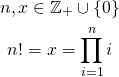If you try to calculate this function for some small values you will notice that the factorial function rises really fast. For instance,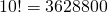. This means that it is no use in considering really big arguments because we will not be able to represent them using casual int32 representation. For our purposes we will assume that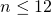.

# Actual formula

Since we know the upper bound forwe can easily implement the formula: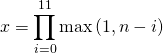This works in the following way: because, we need to multiply at most 12 numbers. In every loop iteration we subtractfromand take the maximum of the difference and one. We do that because we don’t know the value ofand we cannot allow the result to be zeroed. Take a look at this example: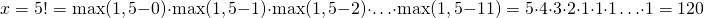For everygreater or equal tothe maximum function returnsso we can be sure that the result is not zeroed. What’s more, we have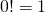as we wanted before.

# Summary

So far we have been using ILP to implement various operations using declarative approach. We have seen that even though ILP uses only trivial tools we are able to implement some fancy functions. We have also seen how to implement strictly imperative construct (if). In the next part we are going to implement another thing from imperative world: loop. Stay tuned.